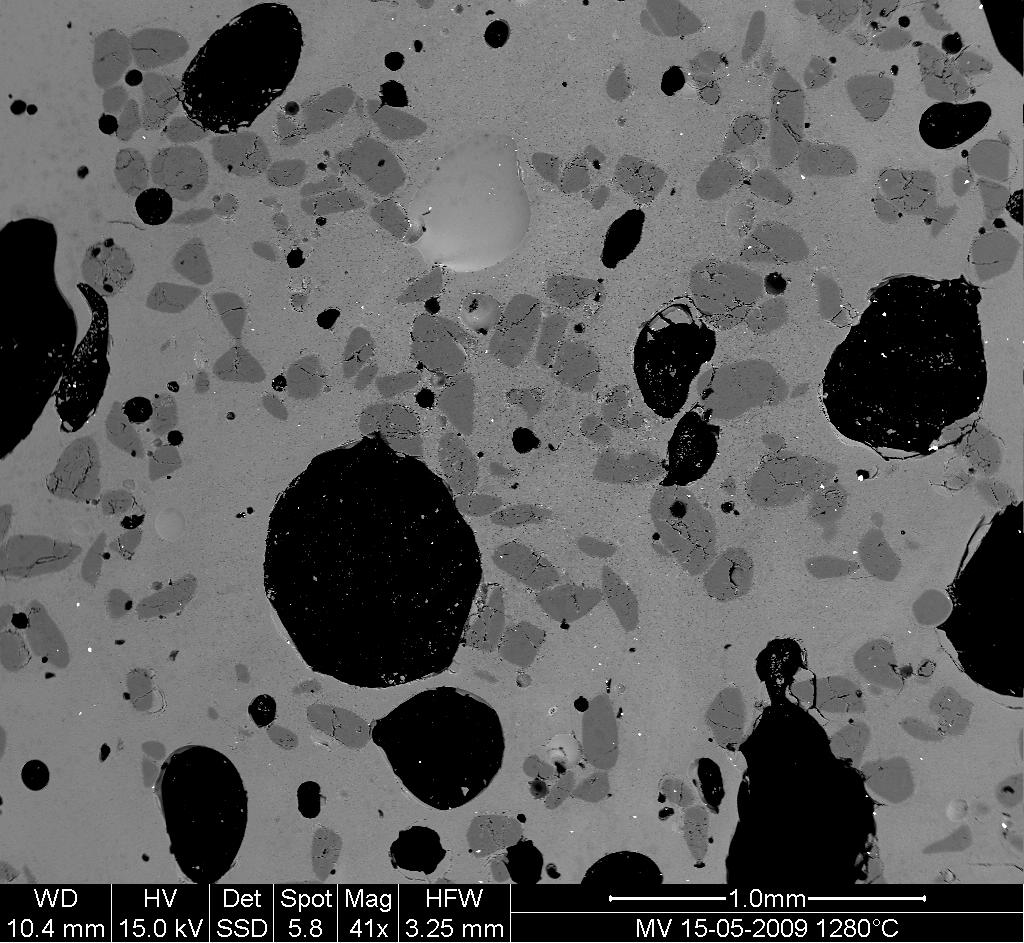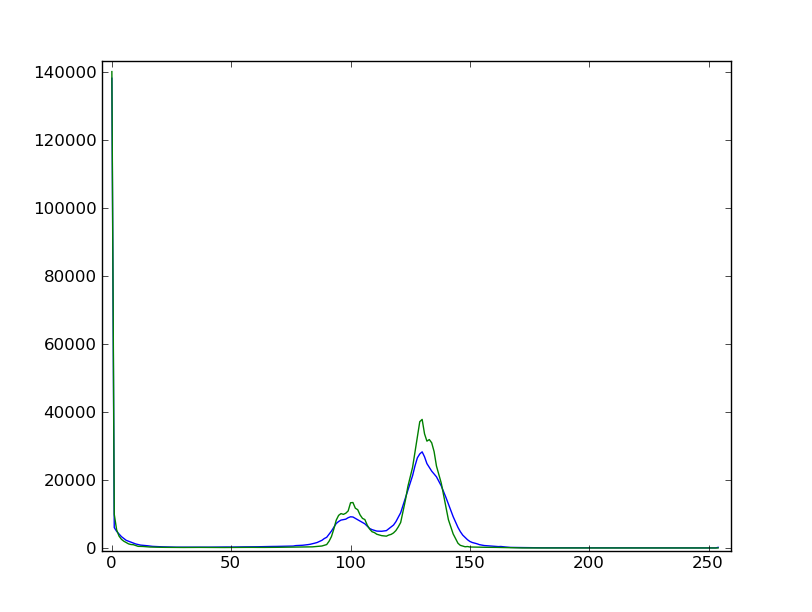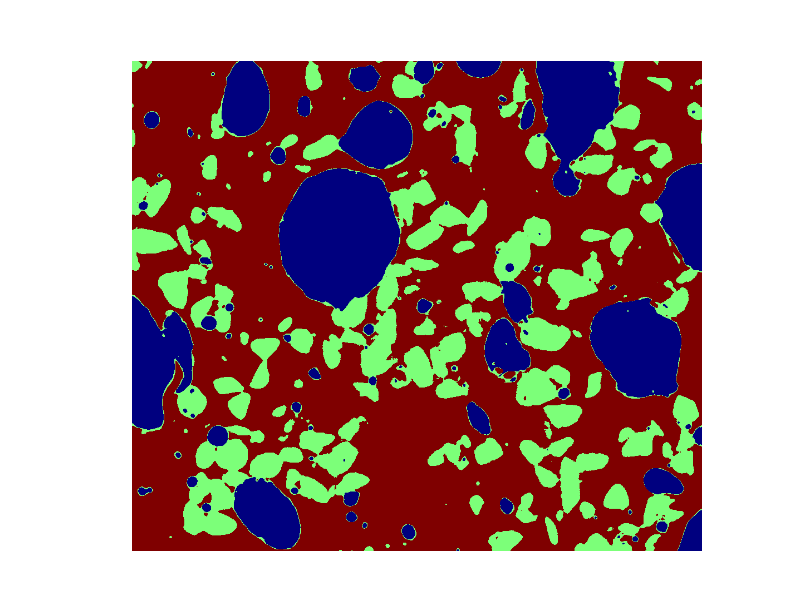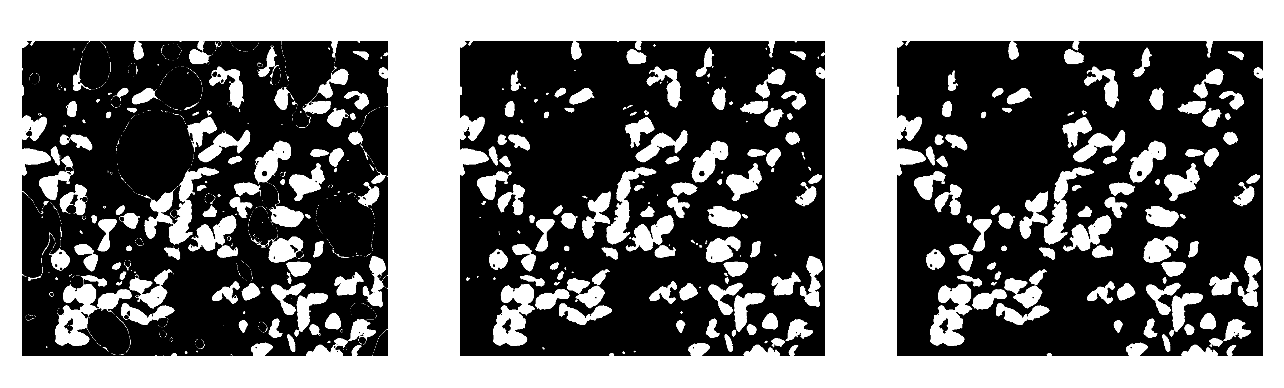```>>> import numpy as np
>>> import matplotlib.pyplot as plt
>>> import scipy as sp
```

# 1.5.11.4. Example of solution for the image processing exercise: unmolten grains in glass¶1. Open the image file MV_HFV_012.jpg and display it. Browse through the keyword arguments in the docstring of `imshow` to display the image with the “right” orientation (origin in the bottom left corner, and not the upper left corner as for standard arrays).

```>>> dat = plt.imread('data/MV_HFV_012.jpg')
```
2. Crop the image to remove the lower panel with measure information.

```>>> dat = dat[:-60]
```
3. Slightly filter the image with a median filter in order to refine its histogram. Check how the histogram changes.

```>>> filtdat = sp.ndimage.median_filter(dat, size=(7,7))
>>> hi_dat = np.histogram(dat, bins=np.arange(256))
>>> hi_filtdat = np.histogram(filtdat, bins=np.arange(256))
```4. Using the histogram of the filtered image, determine thresholds that allow to define masks for sand pixels, glass pixels and bubble pixels. Other option (homework): write a function that determines automatically the thresholds from the minima of the histogram.

```>>> void = filtdat <= 50
>>> sand = np.logical_and(filtdat > 50, filtdat <= 114)
>>> glass = filtdat > 114
```
5. Display an image in which the three phases are colored with three different colors.

```>>> phases = void.astype(int) + 2*glass.astype(int) + 3*sand.astype(int)
```6. Use mathematical morphology to clean the different phases.

```>>> sand_op = sp.ndimage.binary_opening(sand, iterations=2)
```
7. Attribute labels to all bubbles and sand grains, and remove from the sand mask grains that are smaller than 10 pixels. To do so, use `sp.ndimage.sum` or `np.bincount` to compute the grain sizes.

```>>> sand_labels, sand_nb = sp.ndimage.label(sand_op)
>>> sand_areas = np.array(sp.ndimage.sum(sand_op, sand_labels, np.arange(sand_labels.max()+1)))
>>> mask = sand_areas > 100```>>> bubbles_labels, bubbles_nb = sp.ndimage.label(void)